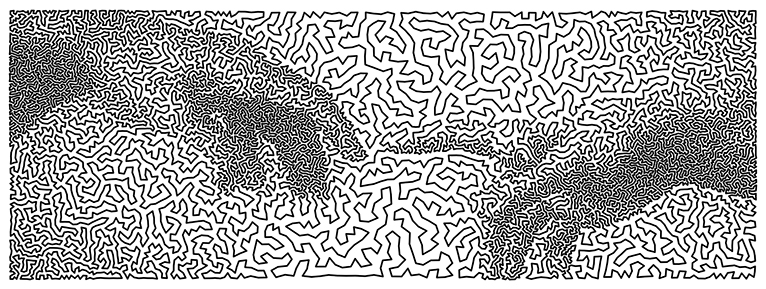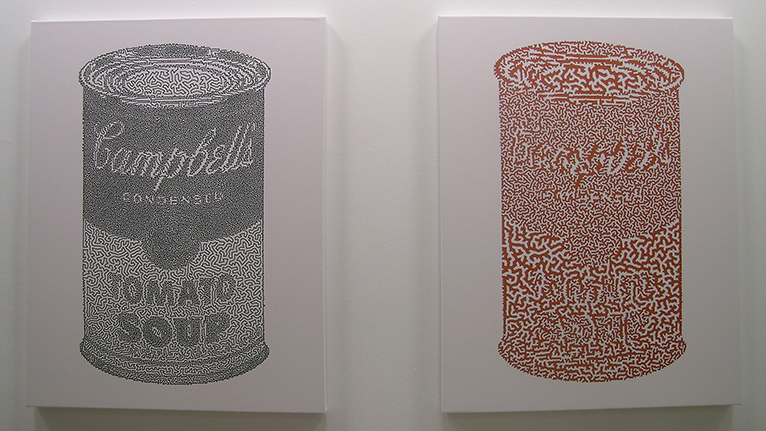# TSP Art and Simple Closed Curves

Is it possible to place a loop of string on sheet of paper in such a way that the string resembles a circle? Yes. A square? Again, yes. But what if we want the string to resemble a portion of Michelangelo's The Creation of Adam? And what if we want the string to be a simple closed curve, a curve that does not intersect itself and has no endpoints, so that when we trace it, we return to our starting point? The answer is once again yes!If you examine the Hands (after Michelangelo) curve shown above, you will find that the curve does not intersect itself. And if you trace it, you will find that you end up where you started. So it is indeed a simple closed curve. This particular simple closed curve was found by solving (but not to optimality) a 12,000-city instance of the Traveling Salesman Problem (TSP).

Mathematicians call simple closed curves Jordan curves after the mathematician Camille Jordan (1838-1922). Jordan's Jordan Curve Theorem states that when a simple closed curve is drawn in the plane, it cuts the plane into two pieces: the part that lies inside the curve, and the part that lies outside it. The artwork shown below, What's Inside? This is!, illustrates the Jordan Curve Theorem. The print on the left shows a simple closed curve that was found by solving (but not to optimality) a 25,000-city instance of the TSP. The print on the right shows the inside of the curve drawn in tomato-soup red.